# Doppler effect

(redirected from Doppler)
Also found in: Dictionary, Thesaurus, Medical, Acronyms, Wikipedia.
Related to Doppler: Doppler effect

## Doppler effect

Doppler effect, change in the wavelength (or frequency) of energy in the form of waves, e.g., sound or light, as a result of motion of either the source or the receiver of the waves; the effect is named for the Austrian scientist Christian Doppler, who demonstrated the effect for sound. If the source of the waves and the receiver are approaching each other (because of the motion of either or both), the frequency of the waves will increase and the wavelength will be shortened—sounds will become higher pitched and light will appear bluer. If the sender and receiver are moving apart, sounds will become lower pitched and light will appear redder. A common example is the sudden drop in the pitch of a train whistle as the train passes a stationary listener. The Doppler effect in reflected radio waves is employed in radar to sense the velocity of the object under surveillance. In astronomy, the Doppler effect for light is used to measure the velocity (and indirectly distance) and rotation of stars and galaxies along the direction of sight. In the spectrum of nearly every star there are wavelengths, characteristic of atoms, that lie near but not quite coincident to the same wavelengths as measured in the laboratory. The small deviations or shifts are generally due to the relative motion of the celestial object and the earth. Both blue shifts and red shifts are observed for various objects, indicating relative motion both toward and away from the earth. Such shifts have been used to measure the orbital velocity of the earth, to detect binary stars and variable stars, and to detect rotation of other galaxies. The Doppler effect is responsible for the red shifts of distant galaxies, and also of quasars, and thus provides the best evidence for the expansion of the universe, as described by Hubble's law. In addition to observations of visible light, the Doppler effect for radio waves is utilized by astronomers to determine the velocities of dust clouds in the spiral arms of the Milky Way galaxy. These observations provided the first direct proof that our own galaxy is rotating. The Doppler shift in radar pulses reflected from the surfaces of Venus and Mercury have been analyzed to obtain new values for their periods of rotation about their axes.

## Doppler effect

The change in the frequency of a wave observed at a receiver whenever the source or the receiver of the wave is moving relative to the other or to the carrier of the wave (the medium). The effect was predicted in 1842 by C. Doppler, and first verified for sound waves in 1845 from experiments conducted on a moving train.

The Doppler effect for sound waves is now a commonplace experience: If one is passed by a fast car or a plane, the pitch of its noise is considerably higher in approaching than in parting. The same phenomenon is observed if the source is at rest and the receiver is passing it. The linear optical Doppler effect was first observed in 1905 from a shift of spectral lines emitted by a beam of fast ions (canal rays) emerging from a hole in the cathode of a gas discharge tube run at high voltage. Still, their velocity was several orders of magnitude below that of light in vacuum. The precise interferometric experiments of A. A. Michelson and E. W. Morley (1887) showed clearly that the velocity of light is not bound to any ether, but is measured to be the same in any moving system. This result was a crucial check for A. Einstein's theory of special relativity (1905), which also makes a clear prediction for the optical Doppler effect.

The Doppler effect has important applications in remote-sensing, high-energy physics, astrophysics, and spectroscopy.

Let a wave from a sound source or radar source, or from a laser, be reflected from a moving object back to the source, which may itself move as well. Then a frequency shift is observed by a receiver connected to the source. The measurement provides an excellent means for the remote sensing of velocities of any kind of object, including cars, ships, planes, satellites, flows of fluids, or winds.

The light from distant stars and galaxies shows a strong Doppler shift to the red, indicating that the universe is rapidly expanding. However, this effect can be mixed up with the gravitational redshift that results from the energy loss which a light quantum suffers when it emerges from a strong gravitational field.

The Doppler width and Doppler shift of spectral lines in sunlight (Fraunhofer lines) are important diagnostic tools for the dynamics of the Sun's atmosphere, indicating its temperature and turbulence.

McGraw-Hill Concise Encyclopedia of Physics. © 2002 by The McGraw-Hill Companies, Inc.

## Doppler effect

A change in apparent frequency (and hence wavelength) of a wave motion as a result of relative motion of the source and observer. For electromagnetic radiation emitted from a moving source, the magnitude of this change is known as the Doppler shift. For a source moving away from the observer, the observed wavelength is longer than it would be if source and observer had no relative motion along the line joining them. This change to longer wavelengths (i.e. toward the red end of the visible spectrum for visible wavelengths) is called a redshift. Conversely, if the source is moving toward the observer, there is a change to shorter wavelengths, i.e. a blueshift.

When there is no relative motion between source and observer, the wavelength of a spectral line can be given by λ. If the relative velocity along the line of sight of source and observer is v , the change in wavelength (δλ) of the spectral line is given by

δλ/λ = v /c

where c is the speed of light. For values of v comparable with c , a relativistic expression is used:

δλ/λ = [(c + v )/(c v )]½ – 1

v is taken to be positive for a receding object and negative for an approaching one. Doppler shifts can be observed in all regions of the electromagnetic spectrum.

The Doppler effect was first suggested by the Austrian physicist C.J. Doppler in 1842. It has proved invaluable in astronomy for measuring the radial velocities of celestial objects and is also used for determining the orbital motions (and in some cases the masses) of spectroscopic binaries and the rotational motions of bodies. See also Doppler broadening.

Collins Dictionary of Astronomy © Market House Books Ltd, 2006
The following article is from The Great Soviet Encyclopedia (1979). It might be outdated or ideologically biased.

## Doppler Effect

a change in the frequency of vibrations or wavelength received by an observer (a vibration receiver) as a result of the motion of the wave source and the observer with respect to one another. The Doppler effect occurs in any wave process of energy propagation. The basic cause of the Doppler effect is a change in the number of waves contained in the propagation path between the source and the receiver. If the length of the waves emitted by the source remains constant, the result is a change in the number of waves reaching the receiver each second, that is, a change in the frequency of the received vibrations.

For elastic waves (sound or seismic waves) and in the general case of electromagnetic waves (light or radio waves) the change in frequency depends on the velocity and direction of the wave source and observer with respect to the medium in which the wave propagates. The propagation of electro-magnetic waves in free space (vacuum) constitutes a special case. In this case the change in frequency is determined solely by the motion of the source and the observer with respect to each other, which is a consequence of Einstein’s principle of relativity.

The Doppler effect in sound waves can be observed directly. It manifests itself in a rise in the pitch of sound as the source and the observer move closer together (that is, as the observer receives more waves per sec). Conversely, the pitch of the sound is lowered as the source and the observer move farther apart.

Let us consider the Doppler effect in the case of monochromatic electromagnetic waves propagating in free space. If the source is stationary with respect to the observer, then in the frame of reference linked with the observer a wave has the same length as in the frame of reference of the source, namely λ0 = c/νo, where c is the velocity of light in a vacuum and νo is the frequency of emitted vibrations. If the source moves uniformly with respect to the observer with a velocity ν directed at an angle a to the beam being observed, then the wavelength in the observer’s frame of reference undergoes a change. Along the beam being observed the change in wavelength is equal to the distance increase over the time 1/νo’ (that is, for one period of emitted vibration):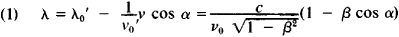In formula (1) λ is the length of the received wave, λ0’ is the length of the emitted wave, and β = v/c. The factortakes into account the time dilatation in the frame of reference of the moving source. As a result of this time dilatation, the measured value of the frequency VQ of the same vibration turns out to be lower in the frame of reference of the observer than in the frame of reference of the source of v0. (This indicates a difference in the rate of time flow between the frame of reference of the moving source and that of the observer; this difference is an effect of the special theory of relativity.)

Equation (1) makes it possible to determine the frequency of vibrations received by an observer,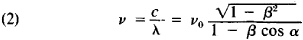A longitudinal Doppler effect occurs if the source moves to-ward the observer (α = 0, cosα = 1) or away from the observer (α = π, cos α = -1):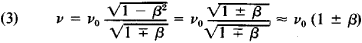The frequency v of the received vibrations increases as the distance between the source and the observer decreases; conversely, this frequency decreases as the distance increases. A longitudinal Doppler effect yields the maximum possible frequency change for a given velocity.

If the source moves around the observer in a circle [α = ±π/2 and cos α = 0 in formula (2)], then in this case the received frequency also differs from the emitted frequency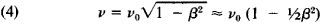even though the number of waves contained in the propagation path remains constant. Formula (4) determines the transverse Doppler effect arising because of the differences in the rate of time flow between the frames of reference of the source and observer. The transverse Doppler effect is an effect of second order of smallness in v/c and is more difficult to observe than the longitudinal effect. There is no transverse Doppler effect in cases where the frequencies are compared in one frame of reference only (as, for instance, in radar).

In those cases where index of refraction n of the medium through which the source is moving has a value other than 1 and is dependent on frequency, the value of the received where n(v) is the index of refraction, dependent on frequency v. In those frequency regions where this dependence is pronounced quite strongly, equation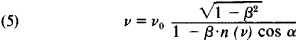where n(v) is the index of refraction, dependent on frequency v. In those frequency regions where this dependence is pronounced quite strongly, equation (5) may have several solutions (complex Doppler effect).

The Doppler effect also arises in mediums whose index of refraction changes with time, even if the source and the receiver are stationary with respect to one another. This phenomenon can be observed in space communications, when a radio beam passes through the earth’s ionosphere, which has a variable index of refraction.

The concept of Doppler effect can be generalized to include changes in the frequency of electromagnetic radiation in a gravitational field (an effect treated in Einstein’s gravitational theory). For instance, a certain line of the sun’s spectrum having a frequency v0’ will be observed on earth as a line having a frequency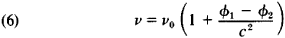where Φ1 and Φ2 are the gravitational potentials of the sun and earth Φ1 Φ1 and Φ2 0). When the radiation of the sun or stars is observed on the earth, the spectral lines shift owing to gravitational effects toward a region of lower frequencies, because ǀΦ1ǀ > ǀΦ2ǀ.

The Doppler effect was named after the Austrian physicist C. Doppler, who in 1842 established the theoretical basis for this effect in acoustics and optics. The Russian physicist V. A. Mikhel’son extended this effect to the case of a medium with variable parameters (in 1899). The existence of a transverse Doppler effect was experimentally confirmed by the American physicists H. Ives and G. Stilwell in 1938.

Almost from the very instant of its discovery the Doppler effect has been used to determine the radial velocities of stars and the rotations of celestial bodies. Investigation of the Doppler shift of the spectral lines of remote galaxies has led to the concept of the expansion of the metagalaxy. Spectroscopic methods make use of the Doppler broadening of spectral lines in the optical region and the radio-frequency region in order to determine the thermal velocities of the atoms and ions in stellar atmospheres and interstellar gas; it is also used to investigate the structure of extragalactic radio sources. In radar and sonar, the Doppler effect is used to determine the velocity of the target. It is also used in space navigation. In radar astronomy it is possible, with the aid of the Doppler effect, to separate the reflections from individual areas of a celestial body having different radial velocities.

### REFERENCES

Landau, L. D., and E. M. Lifshits. Teoriia polia. Moscow, 1967. (Teoreticheskaia fizika, vol. 2.)
Landsberg, G. S. Optika, 4th ed. Moscow, 1957. (Obshchii kurs fiziki; vol. 3.)
Frank, I. M. “Effect Doplera v prelomliaiushchei srede.” Izv. AN SSSR. Seriia fizicheskaia, 1942, nos. 1-2.
Skolnik, M. Vvedeniie v tekhniku radiolokatsionnykh sistem. Moscow, 1965. (Translated from English.)

O. N. RZHIGA

## Doppler effect

[′däp·lər i‚fekt]
(physics)
The change in the observed frequency of an acoustic or electromagnetic wave due to relative motion of source and observer.
McGraw-Hill Dictionary of Scientific & Technical Terms, 6E, Copyright © 2003 by The McGraw-Hill Companies, Inc.

## Doppler effect

The change in frequency with which energy reaches a receiver when the receiver and the energy source are in motion relative to each other. Since the Doppler frequency shift is proportional to radial velocity, a radar system that measures such a shift in frequency can also provide the radial velocity of a target. This effect is also used to separate moving targets from stationary ones even when the undesired clutter power might be much greater than the power of echo from the targets. It is named after Austrian scientist C. J. Doppler. Also called a Doppler shift. *

## Doppler effect

a phenomenon, observed for sound waves and electromagnetic radiation, characterized by a change in the apparent frequency of a wave as a result of relative motion between the observer and the source
Collins Discovery Encyclopedia, 1st edition © HarperCollins Publishers 2005

## Doppler effect

The change in electromagnetic frequency that occurs when the source of the radiation and its observer move toward or away from each other. The faster they come together, the higher the frequency. The faster they move away, the lower the frequency. Discovered by Austrian physicist Christian Doppler (1803-1853), this condition has a great effect on low-earth orbit (LEO) satellites as they weave towards and away from the earth. See Doppler radar.
Copyright © 1981-2019 by The Computer Language Company Inc. All Rights reserved. THIS DEFINITION IS FOR PERSONAL USE ONLY. All other reproduction is strictly prohibited without permission from the publisher.
References in periodicals archive ?
Thus the calculated sensitivity, specificity, PPV and NPV of RI on Doppler USG were 85.4%, 86.4%, 85.4% and 86.4%.
Doppler is a humorous satirical novel that cuts to the quick of what ails modern society.
For that reason, Clark said the station has offered use of the Doppler information to airport officials.
The first influence of Doppler Effect is Delay Spread: Frequency Selective Fading which is about 40 X [10.sup.-6] s [much less than] 2 X [10.sup.-3] s or Ts [much less than] [[sigma].sub.[tau]], where symbol duration is lower than maximum excess delay and (1 MHz [much greater than] 0,1 MHz for MCS 7 and 2 MHz [much greater than] 0,1 MHz for MCS 5) or Bs [much greater than] Bc, where bandwidth of signal is higher than channel bandwidth.
where n denotes the discrete delay series, [[xi].sub.k] is the discrete Doppler frequency, x(m) is the discrete coded signal, and X([omega]) represents the discrete Fourier transform (DFT) of x(m).
Doppler fluctuation is caused by the irregular motions of transmitter or receiver units.
Hence, it has motivated to undertake the present study to evaluate the fetal growth with color Doppler velocimetry in normal and high-risk pregnancies.
Considering the above facts, these six conditions were included in the present study with an aim to evaluate the importance of Doppler study in high-risk pregnancy and to correlate the findings of Doppler with clinical findings in high-risk pregnant women.
The findings of the last UA Doppler at delivery showed that all the patients in group 2 had UA PI >95th percentile for their gestation period, 36 had only raised PI, 16 had AEDF, and 13 had REDF.
Cohorts were divided into normal and abnormal Doppler and compared with respect to both the presence and number of gross and histopathologic findings in the placenta that were plausible in their relation to the FGR.

Site: Follow: Share:
Open / Close